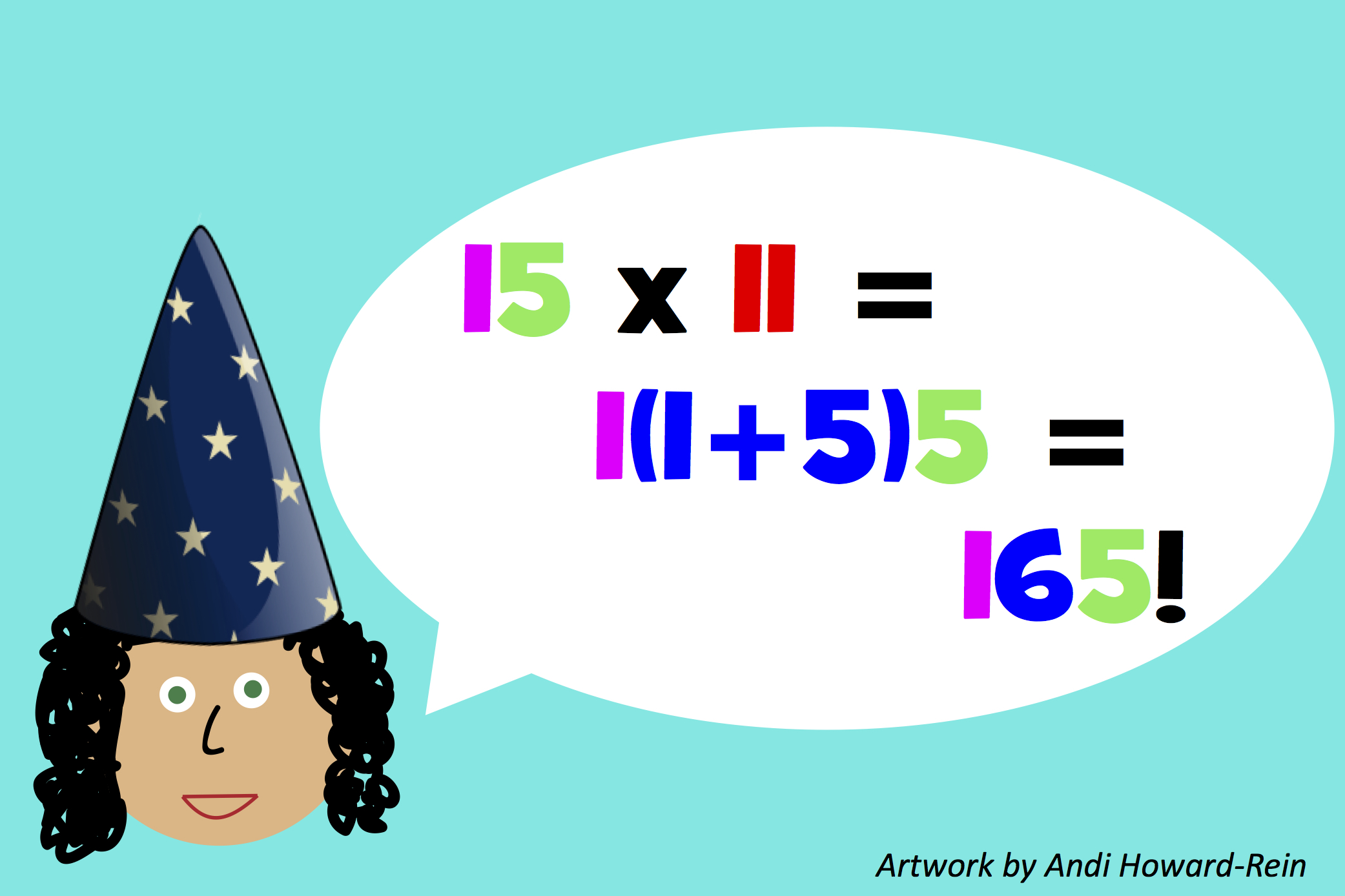# Tricks to Make You a Math WhizNumbers are nice and neat, so they make really cool patterns. If you know those patterns, you can do speedy math tricks in your head. It’s easy to multiply a 2-digit number by 11, and to multiply by 5. You can also “square” numbers ending in 5 (to square means to multiply a number by itself). Let’s learn some of the coolest number tricks out there, so you can wow your family and friends!

Wee ones: Can you count to 5? Try it!

Little kids: What digits do you use to “spell” the number 11? Bonus: What is 11 + 11? And what is that new number plus 11? Try counting up by 11s and see how far you get!

Big kids: To multiply a 2-digit number by 11, you add its two digits and stick that sum in between the digits in the tens place. 32 times 11 is 352, because 3+2=5. So what is 43 x 11?  Bonus: When you multiply by 5, that’s half as much as multiplying by 10. So just cut the number in half, and tack on a zero. Quick, what’s 42 x 5?

The sky’s the limit: To take any 2-digit number ending in 5 and multiply it by itself, you take the first digit, multiply it by the digit 1 more than it, and then just tack 25 onto the end. 35 x 35 is 1225, because 3 x 4 (the number 1 more than 3) is 12, and then you tack on 25…1225. So what’s 55×55?

Wee ones: 1, 2, 3, 4, 5.

Little kids: A 1 and another 1. Bonus: 22, then 33, 44, 55, 66, 77, 88, 99…and then 110!

Big kids: 473, since 4+3=7 and that goes in the tens place.  Bonus: 210. 21 is half of 42, then tack on a zero.

The sky’s the limit: 3025. 5 times the number 1 more than 5 (which is 6) gives you 5 x 6 = 30. Then tack on a 25, to get 3025.

## Pick a Topic

50 States

Animals

Daily Routine

Entertainment

Food

History

Science and Nature

Sports

Vehicles and Transportation